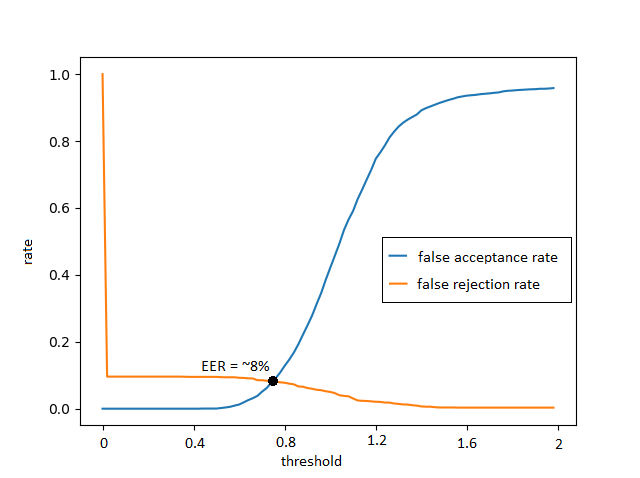# Wierd behaviour of siamese network

Hey,

I’ve adapted Harveyslash solution to siamese network to serve my purpose which is image class verification on custom dataset with transfer learning from network trained to classify this dataset. However I’m stuck on weird behaviour of the network. It’s been several days of diagnosing the problem, but it seems that I’m no closer to figuring it out. Maybe you’ll recognize what’s wrong.

So:
Aim: to train network to verify image class with Siamese architecture (so the network labels pair of images from different classes as dissimilar)

Data set: 5994 classes, 600 grey scale images on average for each class for classification training. For siamese training I’m choosing pairs with varying class number, or pairs per class number (ie. 100 classes, 100 pairs for every class). Pairs are made in a way that approx. half of them is simillar (same classes) and half is dissimilar (different classes). I am labeling similar classes as 0 and dissimilar as 1.

Setup: I’ve trained a ResNet-152 network for classification this data set (60% acc), replaced last fc classification layer with new feature layer of size 512 (`net.fc = nn.Linear(net.fc.in_features, 512)`). I’m using SDG optimizer with momentum 0.9, and I’m using Contrastive Loss as a loss function (from Harveyslash repo):

``````class ContrastiveLoss(torch.nn.Module):
"""
Contrastive loss function.
"""

def __init__(self, margin=2.0):
super(ContrastiveLoss, self).__init__()
self.margin = margin

def forward(self, output1, output2, label):
euclidean_distance = F.pairwise_distance(output1, output2, keepdim=True)
loss_contrastive = torch.mean((1-label.float()) * torch.pow(euclidean_distance, 2) +
(label.float()) * torch.pow(torch.clamp(self.margin - euclidean_distance, min=0.0), 2))

return loss_contrastive

``````

The (simplified) training stage looks like this:

``````    criterion = ContrastiveLoss()
for epoch in range(start_epoch, epochs):
for i_batch, sample_batch in enumerate(trainloader, 0):

# retrieve images and class label fr.om data loader
img_batch_1, class_batch_1 = sample_batch['img1'], sample_batch['class1']
img_batch_2, class_batch_2 = sample_batch['img2'], sample_batch['class2']

img_batch_1 = torch.unsqueeze(img_batch_1, 1).to(device)
img_batch_2 = torch.unsqueeze(img_batch_2, 1).to(device)

# making labels {0, 1} for pairs
label_batch = (class_batch_1 != class_batch_2).to(device)

output1, output2 = net(img_batch_1, img_batch_2)
loss_contrastive = criterion(output1, output2, label_batch)
loss_contrastive.backward()
optimizer.step()
``````

Expected behavior: network output converges to labeling similar as near 0 and dissimilar as near 1
Actual behavior on training set of 100 speakers, 100 pairs: Loss quickly decreases from ~20 to ~1 and persist on this level. On the training set average euclidean distance converges from (before first epoch):
similar pairs: ~12, dissimilar pairs: ~16
to:
similar pairs:~0.9, dissimilar pairs:~1.03
On the eval set average euclidean distance of converges from:
similar: ~5, dissimilar: ~13
to:
similar: ~0.1, dissimilar: ~1.17

So first thing is that interestingly eval set scores waay batter than train set, and the second is that it seems that the network isn’t actually learning anything, but only scales the initial dissimilarity score. I’ve made a diagram with FAR and FRR which gave weird results:I’ve tried freezing all layers except new fc layer, changing learning rate from 0.1 to 0.00001,
What am I doing wrong?

So it turns out that the problem lies in pairwise_distance attribute `keepdim`. Networks learn normally with the attribute set to default (`False`). I’ll dig into that and write why when I’ll have a moment.